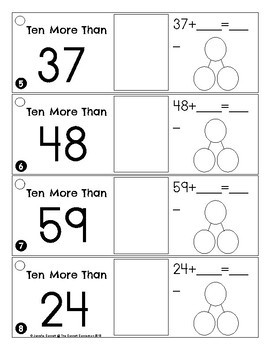# First Grade Math Ten More and Ten LessSubject
Grade Levels
Resource Type
Product Rating
File Type

PDF (Acrobat) Document File

Be sure that you have an application to open this file type before downloading and/or purchasing.

389 KB|31 pages
Share
Also included in:
1. This bundle includes a years worth of math center activities that are each aligned to standards and concepts taught in first grade. These math rings are differentiated to help provided meaningful activities for all levels of learners. These rings can also be used with higher kindergarten students.
\$47.00
\$32.00
Save \$15.00
Product Description
Practice adding ten more and subtracting ten less with these math rings that focus on numbers within 100.

1. Ten More Cards where students must use dry erase markers to draw ten more than the number, fill in the number bond to represent ten more than the number and fill in the number sentence that represents ten more than the number.
2. Ten More Word Problem Cards where students must read the word problem, draw a picture to solve the problem, write a number sentence to solve the problem and write the problem as a number bond.
3. Ten Less Cards where students must use dry erase markers to draw ten less than the number, fill in the number bond to represent ten less than the number and fill in the number sentence that represents ten less than the number.
4. Ten Less Word Problem Cards where students must read the word problem, draw a picture to solve the problem, write a number sentence to solve the problem and write the problem as a number bond.

Simply print the cards and cut apart and laminate. Punch a hole in the top corner and place on a ring for an easily portable center or small group activity. There are optional recording sheets available for each ring.

This product is included in the following bundle:
First Grade Math Ring Bundle

Please check out these other First Grade Math Rings:
Math Rings: Addition by Making 10
Math Rings: Number Bonds for Sums up to 10
Math Rings: One More One Less
Math Rings: Measurements
Total Pages
31 pages
Answer Key
N/A
Teaching Duration
N/A
Report this ResourceSign Up